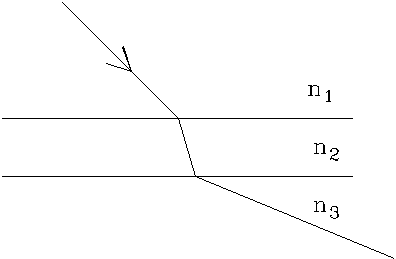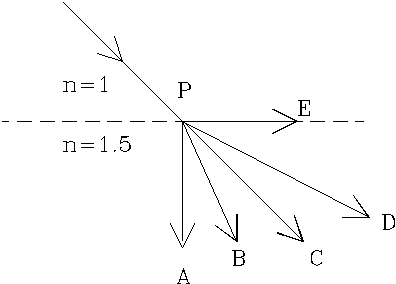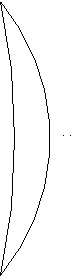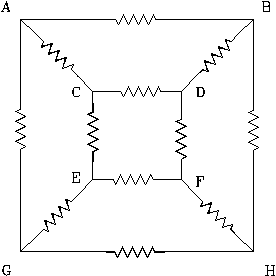SCHOLASTIC APTITUDE PHYSICS 1998

PHYSICS

 Time: ONE Hour Max. Marks: 60

Answers must be written either in English or the medium of instruction of the candidate in high school.

• Answer all the questions in the booklets provided for the purpose.
• There will be no negative marking.
• There are THREE sections. Answer all questions of a section at one place.
• The relevant working in arriving at an answer has to be shown wherever required. However if the answer can be arrived at without detailed working, write only the answer
• Use of calculators or graph papers is not permitted.
• Marks allotted for individual sections are given below. All questions in an individual section carry equal marks
 Section A 10 Marks Section B 20 Marks Section C 30 Marks

Useful Physical constants:

1. Planck’s constant = 6.6 x 10-34 Js
2. Velocity of Light c = 3 x 108 m/s
3. Electron Volt = 1.6 x 10-19 J
4. Relative density of cork = 0.25

SECTION A

1. What temperature change on the Kelvin scale is equivalent to a 10 degree change on the Celsius scale?
A) 283 K B) 273 K C) 18 K D) 10 K E) 0
2. A light year is a unit of
A) acceleration B) distance C) speed D) time E) velocity
3. The critical angle of a material is the angle of incidence for which the angle of refraction is:
A)B) 30° C) 45° D) 90° E) 180°
4. A plane mirror produces an image that is:
A) real, inverted, and larger than the object.
B) real, upright, and the same size as the object.
C) real, upright, and smaller than the object.
D) virtual, upright, and the same size as the object.
5. An object is located 0.20 m from a converging lens which has a focal length of 0.15 m. Relative to the object, the image formed by the lens will be:
A) real, erect, and smaller. B) real, inverted, and smaller.
C) real, inverted, and larger. D) virtual, erect, and larger.
6. If the frequency of sound is doubled, the wavelength:
A) halves and the speed remains unchanged.
B) doubles and the speed remains unchanged.
C) is unchanged and the speed doubles.
D) is unchanged and the speed halves.
7. When metal rod 1 is placed in contact with metal rod 2, thermal energy flows from 1 to 2. A possible explanation is that 1 has a higher
A) specific heat B) heat capacity C) mass D) temperature E) Density
8. When the velocity of a moving object is doubled, another physical quantity associated with the object also gets doubled. This quantity is
A) acceleration B)kinetic energy C) mass D) momentum E) Potential Energy
9. Which of the following could NOT be used to indicate a temperature change? A change in:
A) color of a metal rod. B) length of a liquid column.
C) electrical resistance. D)mass of one mole of gas at constant pressure.
10. Two charges repel each other with a force F when they are a certain distance apart. The charges are halved and the distance between them is also halved. The force between the charges now is
 A) F/16 B) F/4 C) F/8 D)F E) F/2

SECTION B

1. A mass m of helium gas is in a container of constant volume V. It is initially at pressure p and absolute temperature T. Additional helium is added, bringing the total mass of helium gas to 3m. After this addition, the temperature is found to be 2T. What is the gas pressure?
 A) 2 /3 p B) 3 /2 p C) 2 p D) 3 p E) 6 p
2. Which would be the most comfortable temperature for your bath water?
 A) 0o C B) 40 K C) 110o C D) 310 K E) 560 K
3. A wire of length 50 cm has a mass of 20 gm. If it’s radius is halved by stretching, its new mass per unit length will be
 A) 0.4 gm/cm B) 0.2 kg/m C) 2 kg/m D) 0.1 gm/cm E) 0.2 gm/cm
4. A string is tied around a cork of mass m and the free end of the string is attached to the bottom of a water-filled container with the cork totally immersed in the water. The tension in the string is:
 A)0 B) mg C) 2 mg D) 3 mg E) 4 mg
5. A long straight wire conductor is placed below a compass. When the conventional current in the conductor is from south to north and large, the N pole of the compass:
 A) remains undeflected. B) points to the south. C) points to the west. D) points to the east. E) has its polarity reversed.
 A beam of light passes from medium 1 to medium 2 to medium 3 as shown in the accompanying figure. What is true about the respective indices of refraction (n1, n2, and n3)? A) n1 > n2 > n3      B)n1 > n3 > n2 C) n2 > n3 > n1       D)n2 > n1 > n3 E) n3 > n1 > n21. A positively charged conductor attracts a second object. Which of the following statements could be true?
I. The second object is a conductor with negative net charge.
II. The second object is a conductor with zero net charge.
III. The second object is an insulator with zero net charge.
 A) I only B) II only C) III only D) I & II only E) I, II & III
 A ray of light is directed toward a point P on a boundary ( represented by the dotted line ) as shown to the right. Which segment best represents the refracted ray? A) PA                     B) PB C) PC                         D) PD E) PE1. A book is placed on a table. Let FEB be force exerted by earth on the book, FTB be force exerted by table on the book, FBT be force exerted by book on the table and FBE be force exerted by book on the earth. Among these forces, the action, reaction pairs are
 A) FEB , FTB B) FBE , FTB C) FEB , FBE D)FEB , FBT E)FBE , FBT
2. You are given three 1.0 W resistors. Which of the following
equivalent resistances CANNOT be produced using all three resistors?
 A)1 /3 W B) 2 /3 W C) 1.0 W D) 1.5 W E) 3.0 W

SECTION C

1. Two cars are travelling in the same direction at 60 kmph and are 30 km apart. A third car travelling at 30 kmph crosses one of the cars at a certain moment. Find the time elapsed before it crosses the second car. Solve the problem if the first two cars are travelling in the opposite directions.
2. A point object is located at a distance of 30 cm on the principal axis of a convex lens of focal length 20 cm. If the lens is moved away from the object by 10 cm, find the distance through which the image moves.
3. From amongst resistances of 2 W ,3 W ,6 W and 7 W , two resistors are picked and combined first in series and then in parallel. Their equivalent resistances are found to be 2 W and 9 W . Find the parallel equivalent of the remaining two resitances.
 Which of the following is NOT possible for the images formed by the lens in the accompanying figure? A) real and inverted B) real and smaller in size C) real and larger in size D) virtual and erect E) virtual and smaller in size1. A cyclist covers a certain distance in 50 minutes riding his bicycle at a certain speed and in 40 minutes riding at a faster speed. If he rides his bicyle changing his speed from lower to higher speed every ten minutes how long would he take to cover the distance. Consider both the cases that arise.
2. A horse running along a 5 m wide circular track takes p seconds more to travel along the outer edge than the time taken to travel the inner edge. Find the speed of the horse.
3. In a certain process initial energy of the system is -13.6 eV and final energy is - 3.4 eV. The enegy loss is due to the emission of a quantum of light by the system. If the energy of the quantum of light is given by E=hn , where h is the Planck’s constant and n is the frequency of the light, Find the wavelength of light emittted.
4. A metallic sheet of length L, width W and some thickness is melted and formed in to a hollow cylinder. If the inner and outer radii of the cylinder are a and b respectively, and the length of the cylinder is same as the width of the sheet, Find the thickness of the sheet.
5. Two convex lenses of focal lengths 20 cm and 30 cm are placed 50 cm apart coaxially. (i) A point object is located at a distance of 20 cm from the lens of shorter focal length. The rays of light from this object pass through the first lens and then through the second. Find the distance where the rays of light converge from the second lens. (ii) If a parallel beam of light were to be incident on the first lens and the rays refract a second time after first converging, find the distance where the rays converge after second refraction
 In the circuit shown adjacent junctions are connected by equal resistances. The effective resistance between junctions A and G when the resistances between junctions C,E and D,F are removed is R. The removed resistances are reconnected and the resistances between junctions B,D and F,H are now removed. Find the new effective resistance between A and G.